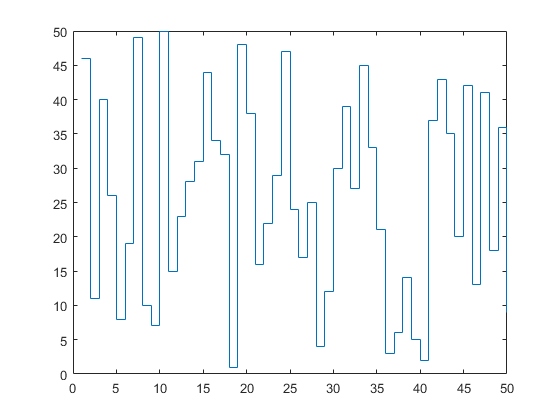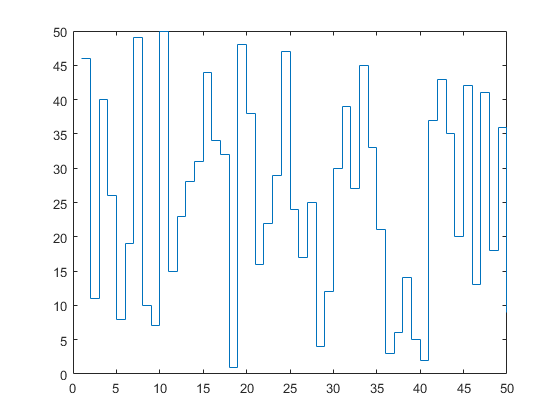## Loren on the Art of MATLABTurn ideas into MATLAB

Note

Loren on the Art of MATLAB has been archived and will not be updated.

# How to Suppress Function Output

You may have noticed that we have some functions that return no output if none is requested, and yet some functions always return something, even if not asked. What's a good way to achieve this in MATLAB?

I do want to point out that while we used to use this programming pattern more often, we have tended to back away from it more recently. For example, you may notice we have two functions for histograms, histogram and histcounts; one for the graphics and one for the computation.

### Contents

#### Example

The function stairs is a prime example.

Compare

array = randperm(50);
stairs(array)with this

s = stairs(array)
%
% You can see that in each case, we get a plot. Only when we ask explicitly
% for an output do we receive one.

s =
Stair with properties:

Color: [0 0.4470 0.7410]
LineStyle: '-'
LineWidth: 0.5000
Marker: 'none'
MarkerSize: 6
MarkerFaceColor: 'none'
XData: [1×50 double]
YData: [1×50 double]

Use GET to show all properties#### Coding Possibility One

In this example, you will see code written the way we used to (and perhaps still do) in MATLAB when we want to return no outputs when called without any output arguments, i.e., when nargout == 0

  function y = attempt1(x)
yy = sin(x);
if nargout > 0 % OR if nargout
y = yy;
end
end

In this case, it's pretty simple. If we want an output, at the end we simply copy the output to the variable with the right output name. If we have many possible output variables, we still need to treat only the first output variable specially.

  function [y,z1,z2,z3] = attempt1a(x)
yy = sin(x);
z1 = yy*17;
z2 = yy+17;
z3 = yy^17;
if nargout > 0 % OR if nargout
y = yy;
end
end

#### Coding Possibility Two

In the second scheme, we write the code with the variable names we want, and then clearvars either just the entire local workspace, or, if we prefer, just the first one, like above.

  function y = attempt2(x)
y = sin(x);
if ~nargout % if nargout == 0
clearvars % or simply clearvars y
end
end

While I hate using functions or commands like clear in function code, I think in this use case, it's okay. When it is NOT okay is when you are trying to coerce the operating system to give up memory. Since MATLAB calls the OS to manage memory, MATLAB is not in control of the timing when the memory it releases actually is available again outside MATLAB. Another technique for clearing memory that's no longer needed is to set the variable(s) whose memory you want reduced to the empty array, e.g., myvar = [].

Do You Have a Preference? Which code pattern to you prefer? Why? Let us know here.

Published with MATLAB® R2019a